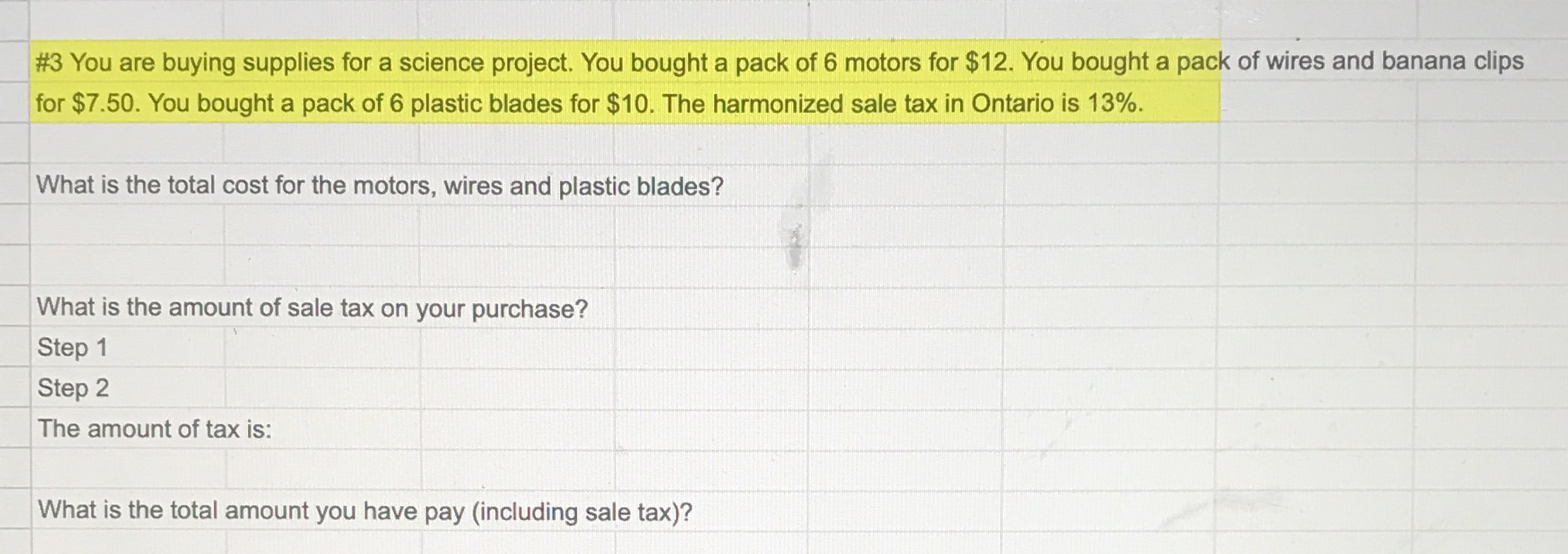### Still have math questions?#3 You are buying supplies for a science project. You bought a pack of $$6$$ motors for $$\ 12$$ . You bought a pack of wires and banana clips for $$\ 7.50$$ . You bought a pack of $$6$$ plastic blades for $$\ 10$$ . The harmonized sale tax in Ontario is $$13 \%$$ . What is the total cost for the motors, wires and plastic blades? What is the amount of sale tax on your purchase? Step $$1$$ Step $$2$$ The amount of tax is: What is the total amount you have pay (including sale tax)?
$$33.335$$